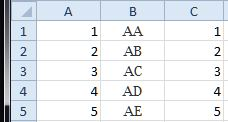## Converting numbers (1, 2, 3…676) to two letters (AA, AB, AC…ZZ) in Excel

Formula 1:

=CHAR(65+TRUNC((A1-1)/26)) & CHAR(65+MOD(A1-1,26))

## Converting two letters (AA, AB, AC…ZZ) to numbers (1, 2, 3…676) in Excel

Formula 2:

=(CODE(MID(B1,1,1))-65)*26 + CODE(MID(B1,2,1))-64

## Example

The figure bellow can be re-created by adding numbers from 1 to 5 in column A. Enter formula 1 in B1 and formula 2 in C1, Copy the formulas in B1 and C1 into the cells below.